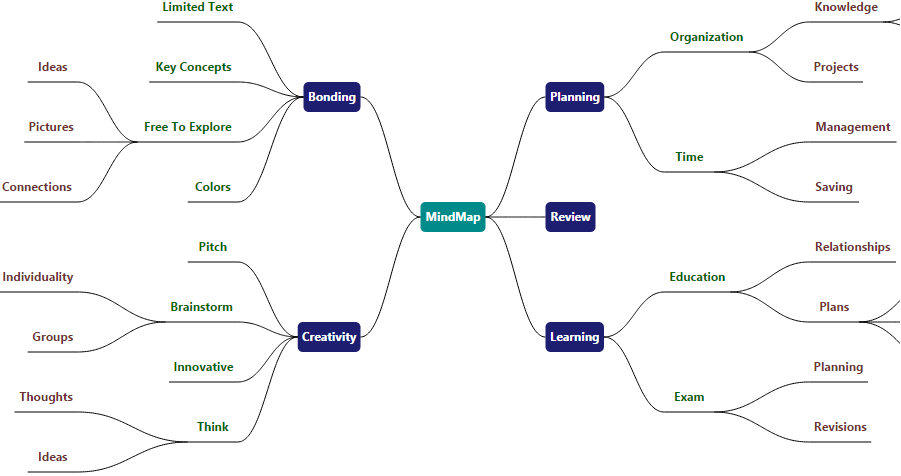# Exporting in JQuery Diagram widget

7 Jun 20194 minutes to read

Diagram provides support to export its content as image/svg files.
The client side method exportDiagram helps to export the Diagram. The following code illustrates how to export the Diagram as image.

• javascript
• ``````var diagram = \$("#diagram").ejDiagram("instance");
//Exports the Diagram as an image of JPEG format
diagram.exportDiagram();``````## Exporting in JQuery Diagram widget options

Diagram provides support to export the desired region of the Diagram to desired formats.

### FileName

FileName is the name of the file to be downloaded. By default, the file name is set as “Diagram”.

### Format

Format is to specify the type/format of the exported file. By default, Diagram is exported as .jpg format. You can export Diagram to the following formats.

• JPG
• PNG
• BMP
• SVG

### Margin

Margin specifies the amount of space that has to be left around the Diagram.

• javascript
• ``````var diagram = \$("#diagram").ejDiagram("instance");
var options = {
fileName: "diagram",
//Margin to the exported file/data
margin: {
left: 30,
right: 30,
top: 30,
bottom: 30
}
};
diagram.exportDiagram(options);``````

### Mode

Mode specifies whether the Diagram is to be exported as files or as data(ImageURL/SVG). The exporting options are as follows.

• Exports Diagram as data of formats ImageURL/SVG

The following code example illustrates how to export the Diagram as raw data.

• javascript
• ``````var diagram = \$("#diagram").ejDiagram("instance");
var options = {
fileName: "diagram",
//Specifies whether to export as files/data
mode: "data"
};
diagram.exportDiagram(options);``````

NOTE

In IE-9, Diagrams cannot be exported as downloadable files. Instead, Diagram provides the exported data (DataURL/SVG) that enables to export it from application.

### Region

You can export any particular region of the Diagram and the region is categorized as follows.

• Region that fits all nodes and connectors that are added to model
• Region that fits all pages (single or multiple pages based on page settings)

The following code example illustrates how to export the region occupied by the Diagram elements.

• javascript
• ``````var diagram = \$("#diagram").ejDiagram("instance");
var options = {
//Specifies the exporting region
mode: "content"
};
diagram.exportDiagram(options);``````

The following code example illustrates how to export any specific region of the Diagram.

• javascript
• ``````var diagram = \$("#diagram").ejDiagram("instance");
var options = {
fileName: "diagram",
//Specifies whether to export as files/data
//Format of the exported file
format: "jpg",
// Defines the custom bounds that has to be exported
bounds: {
x: 1000,
y: 1000,
width: 500,
height: 500
},
};
diagram.exportDiagram(options);``````

### Export Diagram With Stretch option

Diagram provides support to export the diagram as image for stretch option. The exported images will be clearer but larger in file size.

• javascript
• ``````var diagram = \$("#diagram").ejDiagram("instance");
var options = {
fileName: "diagram",
region: "content",
pageWidth: 400,
pageHeight: 400,

//Stretch Option for exporting the Image
stretch: "fill",
multiplePage: false
};
diagram.exportDiagram(options);

//By default, Diagram is exported with stretch option as "none".
The client side method `print` helps to print the Diagram as image. Please refer to below link which shows how to print the Diagram as image and also you can view the several options which can be passed as a parameter in it.